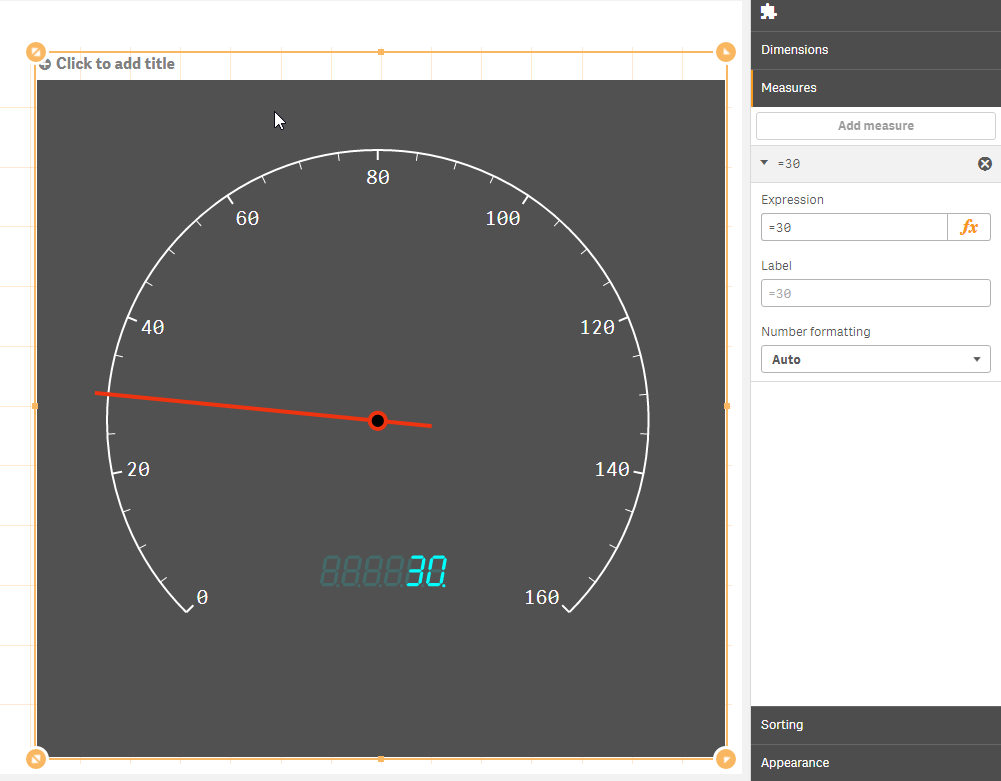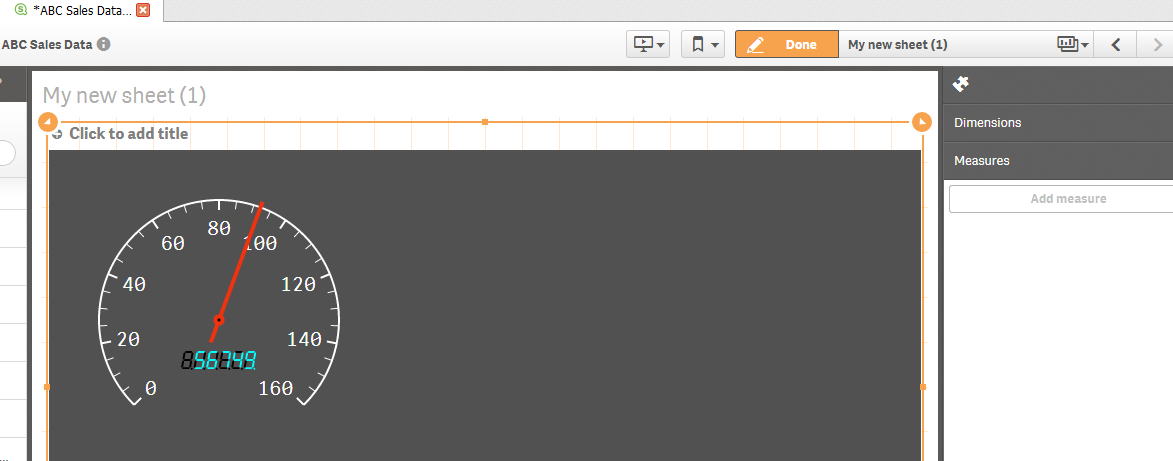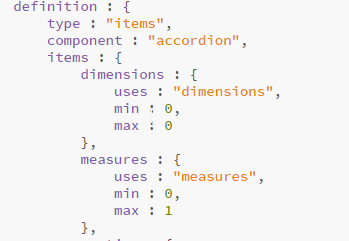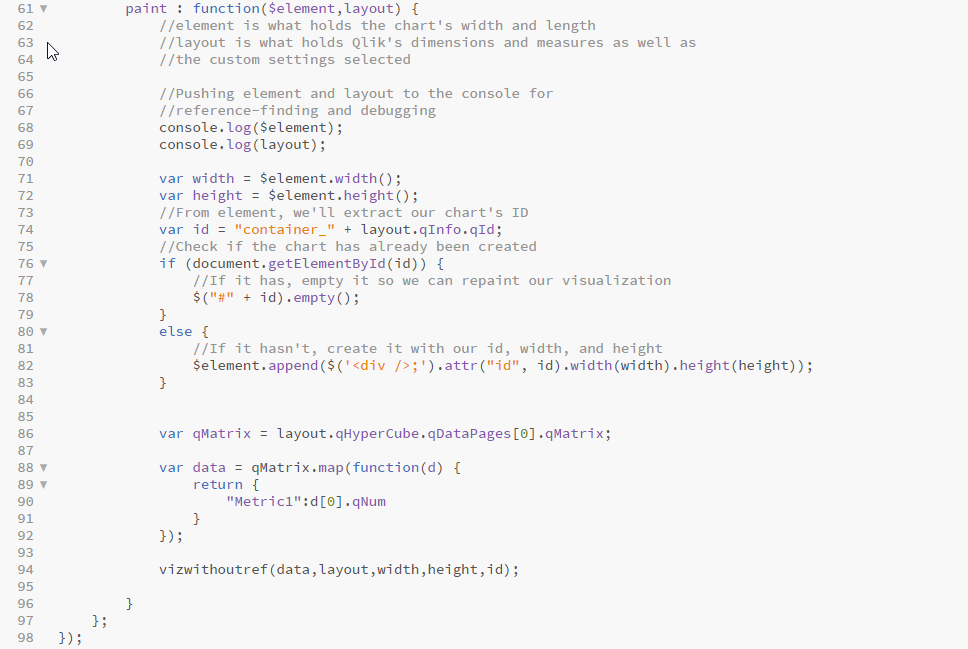# Qlik Sense Enterprise Documents & Videos

Documents & videos about Qlik Sense.

# How to Develop Qlik Sense Extensions from D3.js: Customizing the D3 with Qlik's dataNot applicable

## How to Develop Qlik Sense Extensions from D3.js: Customizing the D3 with Qlik's data

1 - Introduction

2 - Testing the D3 visualization in HTML

3 - Importing the D3 visualization into Qlik Sense

[4 - Customizing the D3 with Qlik's data]

5 - Drawing a second indicator as a reference line [coming soon]

6 - Creating Custom Settings by editing CSS from Javascript [coming soon]

4 - Customizing the D3 with Qlik's Data:

Now that we have our visualization working in Qlik Sense, let's inject Qlik's data in there. After increasing our max/min number of measures and creating a new object, we can add any measure we want, it could be a count, sum, average, of the selected data field. For simplicity, we can add our own number such as "=90" as a measure. We'll start by checking out the measure we just added in the hypercube in layout:Inside layout, we have our extension's qHypercube, that includes the selected data input to the extension, the dimension and measures' labels as well as metadata. The data is divided in data pages. In this extension, we're using only one data page, the initial data page we pulled near the top of our code, Inside each data page in qDataPages is a qMatrix and some metadata about it, such as the number of rows and columns included in this data page. Inside our qMatrix is all the individual rows in the data page. Inside each row, is all the column values for this row. And finally, inside each of these column values is a qNum, a numeric version of the value, and a qText, a textual version of the value. Note that the column values are not defined as a dimension or a measure, it is up to us to use the qText for dimensions and qNums for measures. If we would use the qText of a measure, we would be retrieving the formatted version of the value (for instance 2 decimal places) while the qNum value itself could include many more.

So, we'll start by defining the path to our qMatrix and the data itself (one measure in this case) in variables, so we'll be adding this code snippet to the end of our paint function:

```         var qMatrix = layout.qHyperCube.qDataPages.qMatrix;

var data = qMatrix.map(function(d) {
return {
"Metric1":d.qNum
}
});

```

Now that we have our data ready, let's prepare our extension to receive our data. We'll start by adding more parameters to our function to get access to the data, layout, and the width/height of the object. We'll create a kpi variable that maps to our measure's data in the data variable. Width and height are currently hardcoded in pixels in the first block of code so we'll use the width/height parameters we just added to make the size of the visualization dynamic.

```var vizwithoutref = function(data,layout,width,height,id) {

var kpi = data.map(function(d){return d.Metric1;});

var svg = d3.select("#" + id)
.append("svg")
.attr("width", width)
.attr("height", height);

var gauge = iopctrl.arcslider()
.radius(120)
.events(false)
.indicator(iopctrl.defaultGaugeIndicator);
gauge.axis().orient("in")
.normalize(true)
.ticks(12)
.tickSubdivide(3)
.tickSize(10, 8, 10)
.tickPadding(5)
.scale(d3.scale.linear()
.domain([0, 160])
.range([-3*Math.PI/4, 3*Math.PI/4]));

var segDisplay = iopctrl.segdisplay()
.width(80)
.digitCount(6)
.negative(false)
.decimals(0);

svg.append("g")
.attr("class", "segdisplay")
.attr("transform", "translate(130, 200)")
.call(segDisplay);

svg.append("g")
.attr("class", "gauge")
.call(gauge);
segDisplay.value(kpi);
gauge.value(kpi);
}

```

Don't forget to actually give the function these parameters by adding them in the function call:

```     vizwithoutref(data,layout,width,height,id);

```

Now we can go to Qlik Sense, open DevTools, and refresh the page using F5, change our measure to "=30", and watch our speedometer change from 90 (that we set earlier) to 30.

In order to size the gauge itself correctly however, we could either create a formula that dynamically sizes it based on the width and height, or create a sizing map where we size it a certain way if it's between a certain set of heights and a certain set of widths. In our example, we'll be using a sizing map to keep things clean. If we had gone the formula route, we'd have a hard time finding a formula that fits all kinds of width/height combinations. Since the gauge is circular, we'll be checking if the width or height is shorter and draw the circle's radius using that factor. Once we're done drawing out our sizing map, we can add its variables in the place of hardcoded variables, such as the gauge's radius, gauge's tickPadding, the segDisplay's width, and both the segDisplay and the gauge's tranform statement that tells the D3 library where to paint them in our visualization. Here is our resizable function with the sizing map and the changes in the D3 code:

```var vizwithoutref = function(data,layout,width,height,id) {

var kpi = data.map(function(d){return d.Metric1;});

var widthOrHeight = (width<=height)? width: height;

//Resizing Map Declarations
var fontPercentage = "60%";
var tickPadding = widthOrHeight/200;
var gaugeWidthTransform = -30;
var gaugeHeightTransform = -30;
var displayWidthTransform = ((widthOrHeight/2)*0.8);
var displayHeightTransform = ((widthOrHeight/2)*0.8);

//Resizing Map
if(widthOrHeight<=151){
fontPercentage = "40%";
tickPadding = 1;
gaugeWidthTransform = -37;
gaugeHeightTransform = -35;
displayWidthTransform = ((widthOrHeight/2)*0.825);
displayHeightTransform = ((widthOrHeight/2)*1.45);
}
else if(widthOrHeight>151 && widthOrHeight<=213){
fontPercentage = "50%";
tickPadding = 2;
gaugeWidthTransform = -32;
gaugeHeightTransform = -25;
displayWidthTransform = ((widthOrHeight/2)*0.775);
displayHeightTransform = ((widthOrHeight/2)*1.45);
}
else if(widthOrHeight>213 && widthOrHeight<=276){
fontPercentage = "60%";
tickPadding = 3;
gaugeWidthTransform = -27;
gaugeHeightTransform = -20;
displayWidthTransform = ((widthOrHeight/2)*0.775);
displayHeightTransform = ((widthOrHeight/2)*1.375);
}
else if(widthOrHeight>276 && widthOrHeight<=338){
fontPercentage = "70%";
tickPadding = 4;
gaugeWidthTransform = -20;
gaugeHeightTransform = -9;
displayWidthTransform = ((widthOrHeight/2)*0.8);
displayHeightTransform = ((widthOrHeight/2)*1.375);
}
else if(widthOrHeight>338 && widthOrHeight<=422){
fontPercentage = "80%";
tickPadding = 4;
gaugeWidthTransform = -10;
gaugeHeightTransform = 0;
displayWidthTransform = ((widthOrHeight/2)*0.825);
displayHeightTransform = ((widthOrHeight/2)*1.4);
}
else if(widthOrHeight>422 && widthOrHeight<=504){
fontPercentage = "90%";
tickPadding = 4;
gaugeWidthTransform = 0;
gaugeHeightTransform = 10;
displayWidthTransform = ((widthOrHeight/2)*0.825);
displayHeightTransform = ((widthOrHeight/2)*1.425);
}
else if(widthOrHeight>504){
fontPercentage = "100%";
tickPadding = 4;
gaugeWidthTransform = 20;
gaugeHeightTransform = 20;
displayWidthTransform = ((widthOrHeight/2)*0.825);
displayHeightTransform = ((widthOrHeight/2)*1.4);
}

var svg = d3.select("#" + id)
.append("svg")
.attr("width", width)
.attr("height", height);

var gauge = iopctrl.arcslider()
.radius((widthOrHeight/2)*0.8)
.events(false)
.indicator(iopctrl.defaultGaugeIndicator);
gauge.axis().orient("in")
.normalize(true)
.ticks(12)
.tickSubdivide(3)
.tickSize(10, 8, 10)
.tickPadding(tickPadding)
.scale(d3.scale.linear()
.domain([0, 160])
.range([-3*Math.PI/4, 3*Math.PI/4]));

var segDisplay = iopctrl.segdisplay()
.width((widthOrHeight)/5)
.digitCount(6)
.negative(false)
.decimals(0);

//Preparing transform statements
var transform = "translate(";
var gaugePlacement = transform.concat(gaugeWidthTransform, ",",gaugeHeightTransform,")");
var displayPlacement = transform.concat(displayWidthTransform, ",",displayHeightTransform,")");

//Applying CSS properties and transform statements
svg.append("g")
.attr("class", "gauge")
.attr("transform", gaugePlacement)
.call(gauge);

svg.append("g")
.attr("class", "segdisplay")
.attr("transform", displayPlacement)
.call(segDisplay);

segDisplay.value(kpi);
gauge.value(kpi);
}

```

Now that we've identified the variables in the D3 code that affect the resizing of the visualization, and changed them from being hardcoded to a dynamic variable that we can control, we can go to our app to test the resizing:In the next section, we’ll be modifying the D3 code to add another gauge indicator for a reference line, as well as edit the CSS file for styling our visualization.

Comments
New Contributor II

Hello!

I have been following along with this tutorial up until the point of the qmatrix and have hit a wall. I copied into my java script everything you have up through changing the call script to vizwithoutref(data,layout,width,height,id);

When I open the visualization in Qlik Sense, I am not given an option to edit the measure ="30" (You can see that the measures section is grayed out. I am also attaching my java script file. Please let me know what I am doing wrong. I think it has to do with the calling of my qmatrix variable placement.The link to my .js file:

https://www.dropbox.com/home?preview=speedometerd3twoindicators.jsNot applicable

Hi Nicole,

Thanks for following along. Unfortunately, I can't seem to download the file you linked on dropbox, possibly because you moved the file later? If you can send another link to the file, I can certainly check it out.

One of the things you should check is the initialproperties near the top of the javascript file. If the properties of max measures and min measures are set to 0, that would be the problem:New Contributor II

Thank you Fady. This actually solved the missing measure piece. My next question is where I need to put the hypercube code in my .js script. This code:

var qMatrix = layout.qHyperCube.qDataPages.qMatrix;

var data = qMatrix.map(function(d) {

return

"Metric1":d.qNum

}

});Not applicable

I'm glad that helped, Nicole.

You would put this code snippet in the paint function before you call the visualization function:Contributor

How do I find this layout-viewer that you're referring to?

*Never mind, I found it under Console in the developer tools!

New Contributor III

Very helpful and neatly put post, eagerly waiting for next sections.

Thanks

Raja

Valued Contributor

Hi,

I have changed the max measures to 1 but still the "add measure" is greyed out. Have I done anything wrong? I am doing this in Sense June 2017 desktop.

Thank you very much!

Fei

//Declaring all files we'll be using
define(["jquery",
"text!./speedometerd3twoindicators.css",
"text!./googleplay.css",
"./d3.min",
"./iopctrl",
"./pointergestures",
"./pointerevents"
],
function(\$, cssContent) {

'use strict';
\$("<style>").html(cssContent).appendTo("head");

return {
initialProperties : {
qHyperCubeDef : {
qDimensions : [],
qMeasures : [],
qInitialDataFetch : [{
qWidth : 10,
qHeight : 1000
}]
}
},
//definition is the function that gets called only when
//the extension is created. If you resize or update the
//variables or selections, it doesn't get called again.
definition : {
type : "items",
component : "accordion",
items : {
dimensions : {
uses : "dimensions",
min : 0,
max : 0
},
measures : {
uses : "measures",
min : 0,
max : 1
},
sorting : {
uses : "sorting"
},
//Custom Settings
settings : {
uses : "settings",
items : {
}
}
}
},

snapshot : {
canTakeSnapshot : true
},

//paint is the function that gets called every time the
//variables or selection changes, or if you resize the window.
paint : function(\$element,layout) {
//element is what holds the chart's width and length
//layout is what holds Qlik's dimensions and measures as well as
//the custom settings selected

//Pushing element and layout to the console for
//reference-finding and debugging
console.log(\$element);
console.log(layout);

var width = \$element.width();
var height = \$element.height();
//From element, we'll extract our chart's ID
var id = "container_" + layout.qInfo.qId;
//Check if the chart has already been created
if (document.getElementById(id)) {
//If it has, empty it so we can repaint our visualization
\$("#" + id).empty();
}
else {
//If it hasn't, create it with our id, width, and height
\$element.append(\$('<div />;').attr("id", id).width(width).height(height));
}

var qMatrix = layout.qHyperCube.qDataPages.qMatrix;

var data = qMatrix.map(function(d) {
return {
"Metric1":d.qNum
}
});

vizwithoutref(data,layout,width,height,id);
}
};
});

var vizwithoutref = function(data,layout,width,height,id) {

var kpi = data.map(function(d){return d.Metric1;});

var svg = d3.select("#" + id)
.append("svg")
.attr("width", width)
.attr("height", height);

var gauge = iopctrl.arcslider()
.radius(120)
.events(false)
.indicator(iopctrl.defaultGaugeIndicator);
gauge.axis().orient("in")
.normalize(true)
.ticks(12)
.tickSubdivide(3)
.tickSize(10, 8, 10)
.tickPadding(5)
.scale(d3.scale.linear()
.domain([0, 160])
.range([-3*Math.PI/4, 3*Math.PI/4]));

var segDisplay = iopctrl.segdisplay()
.width(80)
.digitCount(6)
.negative(false)
.decimals(0);

svg.append("g")
.attr("class", "segdisplay")
.attr("transform", "translate(130, 200)")
.call(segDisplay);

svg.append("g")
.attr("class", "gauge")
.call(gauge);
segDisplay.value(kpi);
gauge.value(kpi);

New Contributor III

Hi,

Hope you had refreshed the Qlikense app after the JS Script change, you can cross check the changes using Console.-> Sources.Thanks

RajaLuminary

You have to go out of the app and in again. The dimension/measure  info only gets loaded when you open the app.

New Contributor III

No not required, simple refresh (F5) will do, if Disable Cache is checked as shown.

Show dev tools -> Settings ->  Disable cache (while DevTools is open)Thanks

Raja

Version history
Revision #:
1 of 1
Last update:
‎06-13-2016 02:04 PM
Updated by: